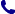• Kontakt
• Newsletter Zapisz się po informacje o nowościach i specjalnych ofertach. Administrator danych: ABE-IPS sp. z o.o.
Wybierz interesujące Cię kategorie
Wyrażam zgodę na przetwarzanie moich danych osobowych w celach marketingowych, tym samym akceptuję Regulamin Newslettera i Politykę prywatności.
Wyrażam zgodę na przesyłanie informacji handlowych drogą elektroniczną.
Wypisz się
• Klienci instytucjonalni
• Dostawa
• O nas
Dostęp on-line

# Książki

0.00 PLN
Schowek (0)
 Schowek jest pusty

## Statistics and Chemometrics for Analytical Chemistry

 Autorzy Wydawnictwo Pearson Education Limited Data wydania 16/04/2018 Liczba stron 312 Forma publikacji książka w miękkiej oprawie Poziom zaawansowania Dla szkół wyższych i kształcenia podyplomowego ISBN 9781292186719 Kategorie Chemia analityczna Zastępuje 9780273730422
253.00 PLN (z VAT)
\$64.07 / €56.55 / £50.69 /
Produkt dostępny
Dostawa 2 dni
Ilość
Do schowka

## Opis książki

Statistics and Chemometrics for Analytical Chemistry 7th edition provides a clear, accessible introduction to main statistical methods used in modern analytical laboratories. It continues to be the ideal companion for students in Chemistry and related fields keen to build their understanding of how to conduct high quality analyses in areas such as the safety of food, water and medicines, environmental monitoring, and chemical manufacturing. With a focus on the underlying statistical ideas, this book incorporates useful real world examples, step by step explanation and helpful exercises throughout. Features of the new edition: * Significant revision of the Quality of analytical measurements chapter to incorporate more detailed coverage of the estimation of measurement uncertainty and the validation of analytical methods. * Updated coverage of a range of topics including robust statistics, Bayesian methods, and testing for normality of distribution, plus expanded material on regression and calibration methods. * Additional experimental design methods, including the increasingly popular optimal designs. * Worked examples have been updated throughout to ensure compatibility with the latest versions of Excel and Minitab. * Exercises are available at the end of each chapter to allow student to check understanding and prepare for exams. Answers are provided at the back of the book for handy reference. This book is aimed at undergraduate and graduate courses in Analytical Chemistry and related topics. It will also be a valuable resource for researchers and chemists working in analytical chemistry.

Statistics and Chemometrics for Analytical Chemistry

## Spis treści

Preface to the seventh edition

Preface to the first edition

Acknowledgements

Glossary of symbols

1 Introduction

1.1 Analytical problems

1.2 Errors in quantitative analysis

1.3 Types of error

1.4 Random and systematic errors in titrimetric analysis

1.5 Handling systematic errors

1.6 Planning and design of experiments

1.7 Statistical calculations

Bibliography and resources

Exercises

2 Statistics of repeated measurements

2.1 Mean and standard deviation

2.2 The distribution of repeated measurements

2.3 Log-normal distribution

2.4 Definition of a 'sample'

2.5 The sampling distribution of the mean

2.6 Confidence limits of the mean for large samples

2.7 Confidence limits of the mean for small samples

2.8 Presentation of results

2.9 Other uses of confidence limits

2.10 Confidence limits of the geometric mean for a log-normal distribution

2.11 Propagation of random errors

2.12 Propagation of systematic errors

Bibliography

Exercises

3 Significance tests

3.1 Introduction

3.2 Comparison of an experimental mean with a known value

3.3 Comparison of two experimental means

3.4 Paired t-test

3.5 One-sided and two-sided tests

3.6 F-test for the comparison of standard deviations

3.7 Outliers

3.8 Analysis of variance

3.9 Comparison of several means

3.10 The arithmetic of ANOVA calculations

3.11 The chi-squared test

3.12 Testing for normality of distribution

3.13 Conclusions from significance tests

3.14 Bayesian statistics

Bibliography

Exercises

4 The quality of analytical measurements

4.1 Introduction

4.2 Sampling

4.3 Separation and estimation of variances using ANOVA

4.4 Sampling strategy

4.5 Introduction to quality control methods

4.6 Shewhart charts for mean values

4.7 Shewhart charts for ranges

4.8 Establishing the process capability

4.9 Average run length: CUSUM charts

4.10 Zone control charts (J-charts)

4.11 Proficiency testing schemes

4.12 Method performance studies (collaborative trials)

4.13 Uncertainty

4.14 Acceptance sampling

4.15 Method validation

Bibliography

Exercises

5 Calibration methods in instrumental analysis: Regression and correlation

5.1 Introduction: instrumental analysis

5.2 Calibration graphs in instrumental analysis

5.3 The product-moment correlation coefficient

5.4 The line of regression of y on x

5.5 Errors in the slope and intercept of the regression line

5.6 Calculation of a concentration and its random error

5.7 Limits of detection

5.8 The method of standard additions

5.9 Use of regression lines for comparing analytical methods

5.10 Weighted regression lines

5.11 Intersection of two straight lines

5.12 ANOVA and regression calculations

5.13 Introduction to curvilinear regression methods

5.14 Curve fitting

5.15 Outliers in regression

Bibliography

Exercises

6 Non-parametric and robust methods

6.1 Introduction

6.2 The median: initial data analysis

6.3 The sign test

6.4 The Wald-Wolfowitz runs test

6.5 The Wilcoxon signed rank test

6.6 Simple tests for two independent samples

6.7 Non-parametric tests for more than two samples

6.8 Rank correlation

6.9 Non-parametric regression methods

6.10 Introduction to robust methods

6.11 Simple robust methods: trimming and winsorisation

6.12 Further robust estimates of location and spread

6.13 Robust ANOVA

6.14 Robust regression methods

6.15 Re-sampling statistics

6.16 Conclusion

Bibliography and resources

Exercises

7 Experimental design and optimisation

7.1 Introduction

7.2 Randomisation and blocking

7.3 Two-way ANOVA

7.4 Latin squares and other designs

7.5 Interactions

7.6 Identifying the important factors: factorial designs

7.7 Fractional factorial designs

7.8 Optimal experimental designs

7.9 Optimisation: basic principles and univariate methods

7.10 Optimisation using the alternating variable search method

7.11 The method of steepest ascent

7.12 Simplex optimisation

7.13 Simulated annealing

Bibliography and resources

Exercises

8 Multivariate analysis

8.1 Introduction

8.2 Initial analysis

8.3 Principal component analysis

8.4 Cluster analysis

8.5 Discriminant analysis

8.6 K-nearest neighbour (KNN) method

8.7 Disjoint class modelling

8.8 Regression methods

8.9 Multiple linear regression (MLR)

8.10 Principal components regression (PCR)

8.11 Partial least-squares (PLS) regression

8.12 Natural computation methods: artificial neural networks

8.13 Conclusion

Bibliography and resources

Exercises

Solutions to exercises

Appendix 1: Commonly used statistical significance tests

Appendix 2: Statistical tables

## Polecamy również książki801 777 223